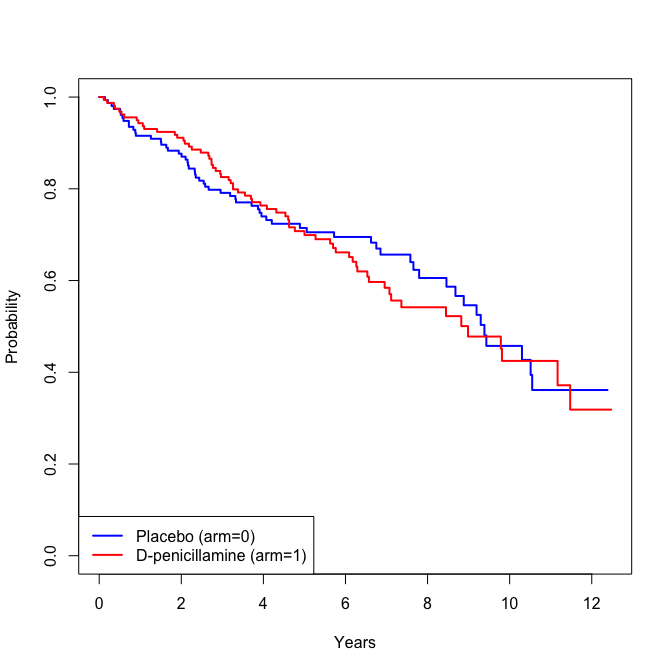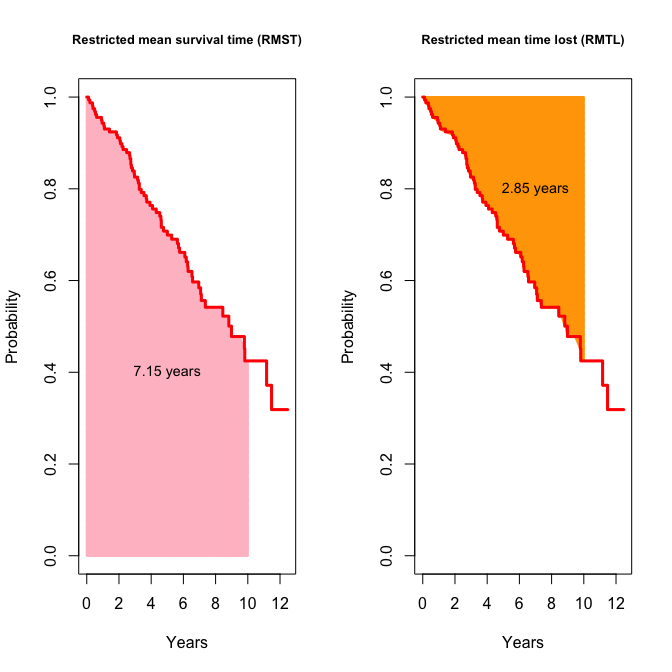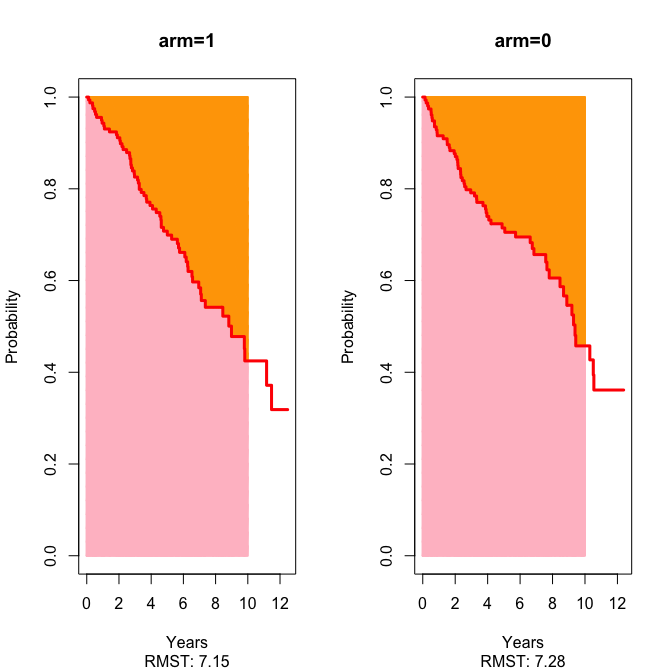# Vignette for survRM2 package: Comparing two survival curves using the restricted mean survival time

## 1 Introduction

In a comparative, longitudinal clinical study, often the primary endpoint is the time to a specific clinical event, such as death, heart failure hospitalization, tumor progression, and so on. The hazard ratio estimate is almost routinely used to quantify the treatment difference. However, the clinical meaning of such a model-based between-group summary can be rather difficult to interpret when the underlying model assumption (i.e., the proportional hazards assumption) is violated, and it is difficult to assure that the modeling is indeed correct empirically. For example, a non-significant result of a goodness-of-fit test does not necessary mean that the proportional hazards assumption is “correct.” Other issues on the hazard ratio is seen elsewhere [1, 2]. Between-group summery metrics based on the restricted mean survival time (RMST) are useful alternatives to the hazard ratio or other model-based measures. This vignette is a supplemental documentation for survRM2 package and illustrates how to use the functions in the package to compare two groups with respect to the restricted mean survival time. The package was made and tested on R version 3.3.2.

## 2 Sample data

Throughout this vignette, we use a part of data from the primary biliary cirrhosis (pbc) study conducted by the Mayo Clinic, which is included in survival package in R. The details of the study and the data elements are seen in the help file in survival package, which can be seen by

library(survival)
?pbc

The original data in the survival package consists of data from 418 patients, which includes those who participated in the randomized clinical trial and those who did not. In the following illustration, we use only 312 cases who participated in the randomized trial (158 cases on D-penicillamine group and 154 cases on Placebo group). The following function in survRM2 package creates the data used in this vignette, selecting the subset from the original data file.

library(survRM2)

D = rmst2.sample.data()
nrow(D)
##  312
head(D[,1:3])
##        time status arm
## 1  1.095140      1   1
## 2 12.320329      0   1
## 3  2.770705      1   1
## 4  5.270363      1   1
## 5  4.117728      0   0
## 6  6.852841      1   0

Here, time is years from the registration to death or last known alive, status is the indicator of the event (1: death, 0: censor), and arm is the treatment assignment indicator (1: D-penicillamine, 0: Placebo). Below is the Kaplan-Meier (KM) estimate for time-to-death of each treatment group.## 3 Restricted mean survival time (RMST) and restricted mean time lost (RMTL)

The RMST is defined as the area under the curve of the survival function up to a time $$\tau (< \infty):$$ $\mu_{\tau} = \int_0^{\tau} S(t)dt,$ where $$S(t)$$ is the survival function of a time-to-event variable of interest. The interpretation of the RMST is that “when we follow up patients for $$\tau,$$ patients will survive for $$\mu_{\tau}$$ on average,” which is quite straightforward and clinically meaningful summary of the censored survival data. If there were no censored observations, one could use the mean survival time $\mu_{\infty} = \int_0^{\infty} S(t)dt,$ instead of $$\mu_{\tau}.$$

A natural estimator for $$\mu_{\tau}$$ is $\hat{\mu}_{\tau} = \int_0^{\tau} \hat{S}(t)dt,$ where $$\hat{S}(t)$$ is the KM estimator for $$S(t).$$ The standard error for $$\hat{\mu}_{\tau}$$ is also calculated analytically; the detailed formula is given in . Note that $$\mu_{\tau}$$ is estimable even under a heavy censoring case. On the other hand, although median survival time, $$S^{-1}(0.5),$$ is also a robust summary of survival time distribution, it will become inestimable when the KM curve does not reach 0.5 due to heavy censoring or rare events.

The RMTL is defined as the area “above” the curve of the survival function up to a time $$\tau:$$ $\tau - \mu_{\tau} = \int_0^{\tau} \{ 1-S(t) \}dt.$ In the following figure, the area highlighted in pink and orange are the RMST and RMTL estimates, respectively, in D-penicillamine group, when $$\tau$$ is 10 years. The result shows that the average survival time during 10 years of follow-up is 7.15 years in the D-penicillamine group. In other words, during the 10 years of follow-up, patients treated by D-penicillamine lost 2.85 years in average sense.### 3.1 Unadjusted analysis and its implementation

Let $$\mu_{\tau}(1)$$ and $$\mu_{\tau}(0)$$ denote the RMST for treatment group 1 and 0, respectively. Now, we compare the two survival curves, using the RMST or RMTL. Specifically, we consider the following three measures for the between-group contrast.

1. Difference in RMST $\mu_{\tau}(1) - \mu_{\tau}(0)$
2. Ratio of RMST $\mu_{\tau}(1) / \mu_{\tau}(0)$
3. Ratio of RMTL $\{ \tau - \mu_{\tau}(1) \} / \{ \tau - \mu_{\tau}(0) \}$

These are estimated by simply replacing $$\mu_{\tau}(1)$$ and $$\mu_{\tau}(0)$$ by their empirical counterparts (i.e.,$$\hat{\mu}_{\tau}(1)$$ and $$\hat{\mu}_{\tau}(0)$$, respectively). For inference of the ratio type metrics, we use the delta method to calculate the standard error. Specifically, we consider $$\log \{ \hat{\mu}_{\tau}(1) \}$$ and $$\log \{ \hat{\mu}_{\tau}(0) \}$$ and calculate the standard error of log-RMST. We then calculate a confidence interval for log-ratio of RMST, and transform it back to the original ratio scale. Below shows how to use the function, rmst2, to implement these analyses.

time   = D$time status = D$status
arm    = D\$arm
rmst2(time, status, arm, tau=10)

The first argument (time) is the time-to-event vector variable. The second argument (status) is also a vector variable with the same length as time, each of the elements takes either 1 (if event) or 0 (if no event). The third argument (arm) is a vector variable to indicate the assigned treatment of each subject; the elements of this vector take either 1 (if active treatment arm) or 0 (if control arm). The fourth argument (tau) is a scalar value to specify the truncation time point $${\bf \tau}$$ for the RMST calculation. Note that $$\tau$$ needs to be smaller than the minimum of the largest observed time in each of the two groups (let us call this the max $$\tau$$). The program will stop with an error message when such $$\tau$$ is specified. When $$\tau$$ is not specified in rmst2, i.e., when the code looks like

rmst2(time, status, arm)

the max $$\tau$$ is used as the default $$\tau.$$ It is always encouraged to confirm that the size of the risk set is large enough at the specified $$\tau$$ in each group to make sure the stability of the KM estimates.

Below is the output with the pbc example when $$\tau=10$$ (years) is specified. The rmst2 function returns RMST and RMTL on each group and the results of the between-group contrast measures listed above.

obj = rmst2(time, status, arm, tau=10)
print(obj)
##
## The truncation time: tau = 10  was specified.
##
## Restricted Mean Survival Time (RMST) by arm
##               Est.    se lower .95 upper .95
## RMST (arm=1) 7.146 0.283     6.592     7.701
## RMST (arm=0) 7.283 0.295     6.704     7.863
##
##
## Restricted Mean Time Lost (RMTL) by arm
##               Est.    se lower .95 upper .95
## RMTL (arm=1) 2.854 0.283     2.299     3.408
## RMTL (arm=0) 2.717 0.295     2.137     3.296
##
##
## Between-group contrast
##                        Est. lower .95 upper .95     p
## RMST (arm=1)-(arm=0) -0.137    -0.939     0.665 0.738
## RMST (arm=1)/(arm=0)  0.981     0.878     1.096 0.738
## RMTL (arm=1)/(arm=0)  1.050     0.787     1.402 0.738

In the present case, the difference in RMST (the first row of the “Between-group contrast” block in the output) was -0.137 years. The point estimate indicated that patients on the active treatment survive 0.137 years shorter than those on placebo group on average, when following up the patients 10 years. While no statistical significance was observed (p=0.738), the 0.95 confidence interval (-0.665 to 0.939) was relatively tight around 0, suggesting that the difference in RMST would be at most +/- one year.

The package also has a function to generate a plot from the rmst2 object. The following figure is automatically generated by simply passing the resulting rmst2 object to plot() function after running the aforementioned unadjusted analyses.

plot(obj, xlab="Years", ylab="Probability")### 3.2 Adjusted analysis and implementation

In most of the randomized clinical trials, an adjusted analysis is usually included in one of the planned analyses. One reason would be that adjusting for important prognostic factors may increase power to detect a between-group difference. Another reason would be we sometimes observe imbalance in distribution of some of baseline prognostic factors even though the randomization guarantees the comparability of the two groups on average. The function, rmst2, in this package implements an ANCOVA type adjusted analysis proposed by Tian et al. , in addition to the unadjusted analyses presented in the previous section.

Let $$Y$$ be the restricted mean survival time, and let $$Z$$ be the treatment indicator. Also, let $$X$$ denote a $$q$$-dimensional baseline covariate vector. Tian’s method consider the following regression model, $g\{ E(Y \mid Z, X) \} = \alpha + \beta Z + \gamma^\prime X,$ where $$g(\cdot)$$ is a given smooth and strictly increasing link function, and $$(\alpha, \beta, \gamma^\prime)$$ is a $$(q+2)$$-dimension unknown parameter vector. Prior to Tian et al. , Andersen et al.  also studied this regression model and proposed an inference procedure for the unknown model parameter, using a pseudo-value technique to handle censored observations. Program codes for their pseudo-value approach are available on the three major platforms (Stata, R and SAS) with detailed documentation [6, 7]. In contrast to Andersen’s method [5, 6, 7], Tian’s method  utilizes an inverse probability censoring weighting technique to handle censored observations. The function, rmst2, in this package implements this method.

As shown below, for implementation of Tian’s adjusted analysis for the RMST, the only the difference is if the user passes covariate data to the function. Below is a sample code to perform the adjusted analyses.

rmst2(time, status, arm, tau=10, covariates=x)

where covariates is the argument for a vector/matrix of the baseline characteristic data, x. For illustration, let us try the following three baseline variables, in the pbc data, as the covariates for adjustment.

x=D[,c(4,6,7)]
head(x)
##        age bili albumin
## 1 58.76523 14.5    2.60
## 2 56.44627  1.1    4.14
## 3 70.07255  1.4    3.48
## 4 54.74059  1.8    2.54
## 5 38.10541  3.4    3.53
## 6 66.25873  0.8    3.98

The rmst2 function fits data to a model for each of the three contrast measures (i.e., difference in RMST, ratio of RMST, and ratio of RMTL). For the difference metric, the link function $$g(\cdot)$$ in the model above is the identity link. For the ratio metrics, the log-link is used. Specifically, with this pbc example, we are now trying to fit data to the following regression models:

1. Difference in RMST $E(Y \mid arm,\ X) = \alpha + \beta (arm) + \gamma_1 (age) + \gamma_2(bili) + \gamma_3(albumin),$
2. Ratio of RMST $\log \{ E(Y \mid arm, \ X) \} = \alpha + \beta (arm) + \gamma_1 (age) + \gamma_2(bili) + \gamma_3(albumin),$
3. Ratio of RMTL $\log \{ \tau - E(Y \mid arm, \ X) \} = \alpha + \beta (arm) + \gamma_1 (age) + \gamma_2(bili) + \gamma_3(albumin).$

Below is the output that rmst2 returns for the adjusted analyses.

rmst2(time, status, arm, tau=10, covariates=x)
##
## The truncation time: tau = 10  was specified.
##
## Summary of between-group contrast (adjusted for the covariates)
##                        Est. lower .95 upper .95     p
## RMST (arm=1)-(arm=0) -0.210    -0.883     0.463 0.540
## RMST (arm=1)/(arm=0)  0.968     0.877     1.068 0.514
## RMTL (arm=1)/(arm=0)  1.035     0.806     1.329 0.786
##
##
## Model summary (difference of RMST)
##             coef se(coef)      z     p lower .95 upper .95
## intercept  2.743    2.134  1.285 0.199    -1.440     6.927
## arm       -0.210    0.343 -0.613 0.540    -0.883     0.463
## age       -0.069    0.018 -3.900 0.000    -0.103    -0.034
## bili      -0.325    0.039 -8.386 0.000    -0.401    -0.249
## albumin    2.550    0.472  5.401 0.000     1.624     3.475
##
##
## Model summary (ratio of RMST)
##             coef se(coef)      z     p exp(coef) lower .95 upper .95
## intercept  1.369    0.356  3.842 0.000     3.930     1.955     7.899
## arm       -0.033    0.050 -0.652 0.514     0.968     0.877     1.068
## age       -0.009    0.003 -3.410 0.001     0.991     0.985     0.996
## bili      -0.087    0.013 -6.523 0.000     0.917     0.893     0.941
## albumin    0.360    0.080  4.491 0.000     1.434     1.225     1.678
##
##
## Model summary (ratio of time-lost)
##             coef se(coef)      z     p exp(coef) lower .95 upper .95
## intercept  1.992    0.695  2.865 0.004     7.332     1.876    28.655
## arm        0.035    0.127  0.272 0.786     1.035     0.806     1.329
## age        0.025    0.007  3.810 0.000     1.026     1.012     1.039
## bili       0.063    0.008  8.334 0.000     1.065     1.049     1.080
## albumin   -0.750    0.149 -5.033 0.000     0.472     0.353     0.633

The first block of the output is a summary of the adjusted treatment effect. Subsequently, a summary for each of the three models are provided.

## 4 Conclusions

The issues of the hazard ratio have been discussed elsewhere and many alternatives have been proposed, but the hazard ratio approach is still routinely used. The restricted mean survival time is a robust and clinically interpretable summary measure of the survival time distribution. Unlike median survival time, it is estimable even under heavy censoring. There is a considerable body of methodological research about the restricted mean survival time as alternatives to the hazard ratio approach. However, it seems those methods have been rarely used in practice. A lack of user-friendly, well-documented program with clear examples would be a major obstacle for a new, alternative method to be used in practice. We hope this vignette and the presented survRM2 package will be helpful for clinical researchers to try moving beyond the comfort zone - the hazard ratio.